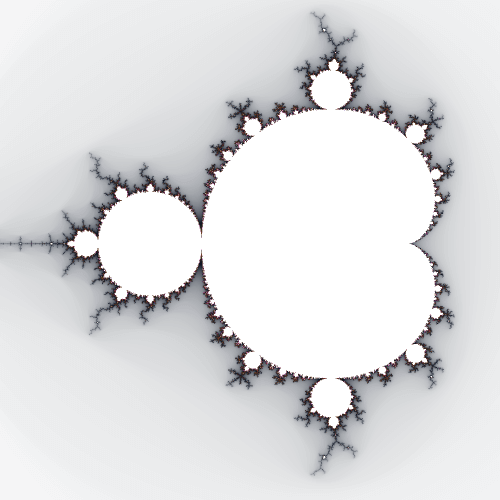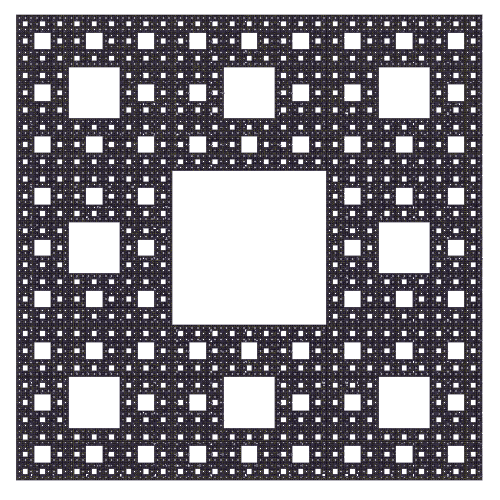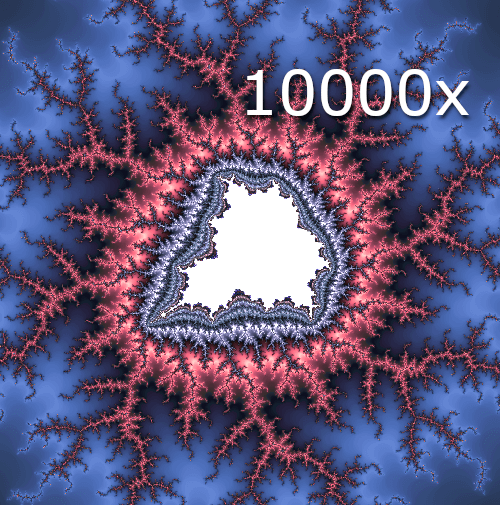# What are Fractals?

What is the definition of Fractal and what are its main features

## Fractals are geometric shapes

They are automatically generated by computer. To generate Fractals they make use special computation through mathematical formula.

These formulas can be very simple too, however they can make unpredictable and awesome results.## Many Fractals are self similar

Fractals contains shapes that are smaller than the main figure. These repetitions can be inifinite.

For instance a square that contains many smaller squares of the same or similar shape.## And lastly, Fractals have an infinite depth

Fractals are not simple images. In fact, they become more and more detailed and unique, increasing the zoom.

Nevertheless, computers haven't got an infinite computational performance. The computer can't compute the image correctly when the zoom is too high.